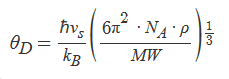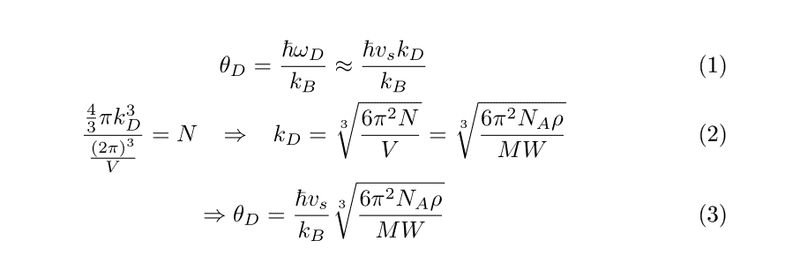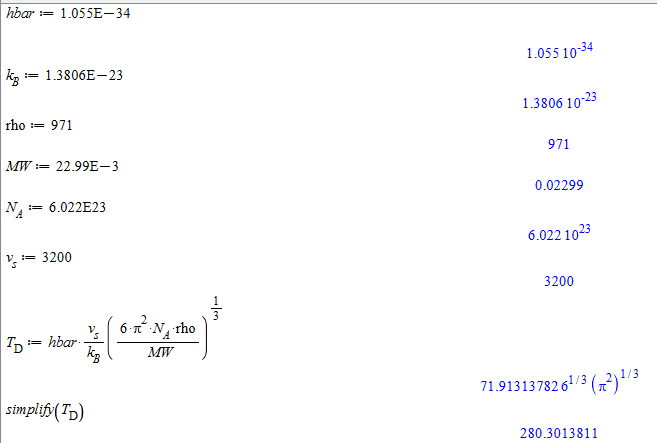# Debye temperature for Na

## Homework Statement

Na has a bcc structure with molecular mass of 22.99 gr/mol, mass density of 0.971 gr/cm^3.
The average speed of sound in Na (at room temperature=300K) is 3200 m/s.
Calculate the Debye temperature for Na

## Homework Equations

I worked out this equation to calculate the Debye temperature (If needed I can show how)## The Attempt at a Solution

I plugged in all the numbers in the above equation with the correct units and I get that the Debye temperature is 280.3K. However, in literature I found that it is approximately 150K.
Is there something wrong with my calculations? Am I missing out on something here?

Thank you!

SteamKing
Staff Emeritus
Homework Helper

## Homework Statement

Na has a bcc structure with molecular mass of 22.99 gr/mol, mass density of 0.971 gr/cm^3.
The average speed of sound in Na (at room temperature=300K) is 3200 m/s.
Calculate the Debye temperature for Na

## Homework Equations

I worked out this equation to calculate the Debye temperature (If needed I can show how)## The Attempt at a Solution

I plugged in all the numbers in the above equation with the correct units and I get that the Debye temperature is 280.3K. However, in literature I found that it is approximately 150K.
Is there something wrong with my calculations? Am I missing out on something here?

Thank you!SteamKing
Staff Emeritus
Homework HelperAnyone?

SteamKing
Staff Emeritus